### What are your chances of acceptance?

Duke University
UCLA
Unweighted GPA: 3.7
1.0
4.0
SAT: 720 math
200
800
| 800 verbal
200
800

#### Extracurriculars

Low accuracy (4 of 18 factors)Michelle Foley
13

# 15 Hardest ACT Math Questions to Improve Your Score

### Do you know how to improve your profile for college applications?

See how your profile ranks among thousands of other students using CollegeVine. Calculate your chances at your dream schools and learn what areas you need to improve right now — it only takes 3 minutes and it's 100% free.

## What’s Covered:

Having a solid grasp of the ACT’s most difficult concepts is a fantastic way to prepare for the test. For this post, we’ve selected some of the hardest questions from official practice tests to help you understand how to solve them. Questions tend to get “harder” and more time-consuming as the test progresses, but hard” is a little subjective; we think these questions are difficult based on working with many students, but you may find some of them easy. Some students will be glad to learn that unlike the SAT, the ACT allows for students to use their calculator on all sections of the math test.

Though we don’t have the space to showcase every single potential problem here, the comprehensive variety of questions provided will leave you well-equipped. Because these questions were selected from official ACT practice tests, they’ll be rather representative of what you’ll be facing on test day.

For each question below, we’ve identified the key concepts being tested and whether a calculator is allowed. We suggest you try solving these on your own before looking at the answer and our suggested solution. Remember: we’ve given you just one way to solve the problem. Most problems can be solved in many ways!

## How do ACT Scores Impact Your College Chances?

Most colleges use the ACT or SAT as a predictor of college success. In fact, some colleges use test scores and grades to filter out applicants. If your academic profile doesn’t meet their expectations, the rest of your application might not be read.

To see how your ACT score impacts your chances of acceptance, try our free Chancing Engine. It will help you predict your odds, let you know how you stack up against other applicants, and suggest aspects of your profile to improve. Unlike other solely stats-based chancing calculators, ours considers much of your entire profile, including both your quantitative stats and qualitative extracurriculars.## Math Topics on the ACT

Before we go into the questions, we want you to understand the terms that the ACT uses to categorize the topics. We’ve also included how many questions fall under each category, so if you’re self-studying, you can prioritize the types of questions that appear more often.

### Preparing for Higher Math (57-60%)

Most recent mathematics that students are learning up to the beginning of 12th grade, starting with algebraic expression and solutions. This category is divided into the following five subcategories:

#### Number and Quantity (7-10%)

Real and complex number systems. Reason with integer and rational exponents, vectors and matrices, and more.

#### Algebra (12-15%)

Solve, graph, and model expressions in many forms, including linear, polynomial, radical, and exponential ones. Students will reason with multiple matrices.

#### Functions (12-15%)

Manipulate, translate, and reason with function definition, notation, representation, and application. Questions may include but are not limited to linear, radical, piecewise, polynomial, and logarithmic functions. Students will find and apply important graph features.

#### Geometry (12-15%)

Reason with congruence and similarity relationships, surface area and volume measurements, and other information regarding shapes and solids. Solve for missing values in triangles, circles, and other figures. Use trigonometric ratios and equations of conic sections.

#### Statistics and Probability (8-12%)

Calculate probabilities, work with distribution, utilize data collection methods, reason with relationships in bivariate data.

### Integrating Essential Skills (40-43%)

These concepts are ones typically learned before the 8th grade, including rates, percentages, proportional relationships, area, surface area, volume, average/median, and expressing numbers in different ways

### Modeling (<25%)

Produce, interpret, understand, evaluate, and improve models. This category measures modeling skills across varied mathematical topics, including those listed above.

## 15 Hardest Math ACT Questions

### Question 1: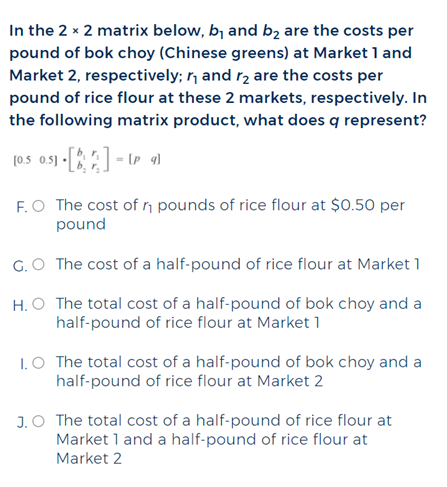Category: Number and Quantity

Here’s how to solve it:

• If you’re unfamiliar with matrices, this is your friendly reminder to brush up on them! In this problem, we will use a method called matrix multiplication. Under this method, the number of columns in the first matrix equals the number of rows in the second. For this question, we’ll refer to the products of matrices $$B$$ and $$R$$ as $$BR$$.

• The products of two matrices are found by multiplying rows by columns. More specifically, we will be multiplying a matrix from the row on the left $$(P)$$ with the columns of the matrices on the right $$(Q)$$.

• To solve for $$p$$, let’s start by multiplying the first item in the row, $$0.5$$, by the first item in the column, $$b_1$$.  Then, we multiply the second item in the row, $$0.5$$, by the second item in the column, $$b_2$$. So, $$p= 0.5\:\times\:b_1 + 0.5\:\times\:b_2$$

• Let’s use this exact same method for finding $$q$$, which is the value we actually need to understand for this question (we technically didn’t need to find $$p$$, but we did anyways to illustrate how matrix multiplication works. $$q=0.5\:\times\:r_1 + 0.5\:\times\: r_2$$

• From the equation $$0.5\:\times\:r_1 + 0.5\:\times\:r_2 = q$$, we can draw the conclusion that we bought $$0.5$$ pounds of rice powder from both Market 1 and Market 2, which matches Solution J, which claims that $$q$$ represents “the total cost of a half-pound of rice flour at Market 1 and a half-pound of rice flour at Market 2.”

### Question 2: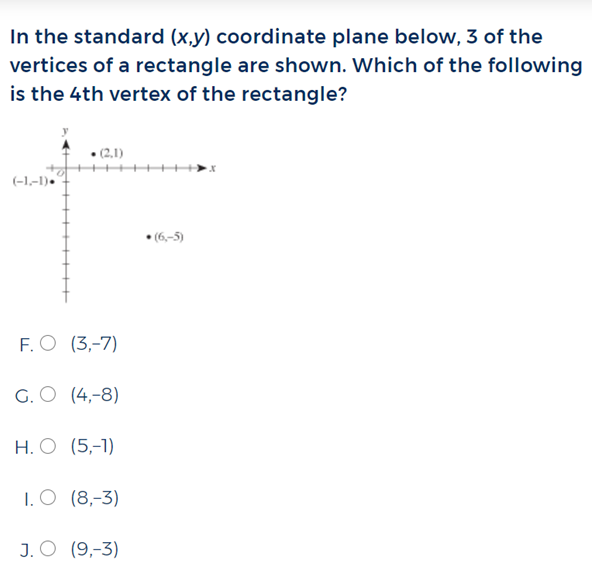Category: Number and Quantity

Here’s how to solve it:

• Questions like these are a mere matter of calculating slope. Know that since the sides of a rectangle are parallel, the slope between $$(-1, -1)$$ and $$(2, 1)$$ will be the same as the one between the fourth vertex and $$(6, -5)$$. We can use this information to calculate the value we need.

• As shown by this equation, one must move up 2 units and right 3 units to travel from point $$(-1, -1)$$ to $$(2, 1)$$:

$$1 – (-1) = 2$$

$$2 – (-1) = 3$$

Therefore, the slope of the shorter sides of this rectangle is $$\frac{3}{2}$$.

• We can use this slope as a formula to apply to $$(6, -5)$$ to find the fourth vertex:

$$x$$ value: $$64 – 3 = 3$$

$$y$$ value: $$-5 – 2 = -7$$

Therefore, the fourth vertex is located at $$(3, -7)$$.

### Question 3:Category: Algebra

Here’s how to solve it:

• To solve this problem, you must first understand what it is asking for. Since we are looking for the difference between the two unnamed numbers, we will start by assigning the variable $$y$$ to the lesser number and $$x$$ to the greater number.

• Now our known information can be quite easily expressed within a pair of algebraic equations:

$$x + y = 151$$

$$y = \sqrt{x} + 19$$

•  To find our values, let’s solve for $$x$$ in terms of $$y$$ in our second equation and substitute it into our first equation:

$$y-19 = \sqrt{x}$$

$$(y-19)^2=x$$

$$(y-19)^2+y=151$$ (first equation)

$$y^2-38y+361+y=151$$

$$y^2-37y+210=0$$

• Since this is a quadratic equation, we will now find the values, or “zeroes” of $$x$$. This can be done on a graphing calculator, but you can also factor. If you recognize that $$210 = 30\:\cdot\:7$$ and $$30+7=37$$, it’s easy to see how the equation should factor.

$$(y-30)(y-7)=0$$

$$y=30, 7$$

• We can then proceed to plug these values into both equations $$a$$ and $$b$$ to see which is best:

For $$y = 7$$ in equation $$b$$:

$$7-19=\sqrt{x}$$

This is not possible because we’d get a negative number equaling $$\sqrt{x}$$.

For $$y = 30$$:

$$30-19=\sqrt{x}$$

$$11=\sqrt{x}$$

$$x= 11^2=121$$

• Therefore, $$x= 121$$ and $$y= 30$$. $$121 – 30 = 91$$, so our answer is J!
• Keep in mind that this type of problem can be solved in many ways. It’s a substitution problem, meaning that you should arrive at the same answer as long as you follow the basic substitution methodologies demonstrated here. Questions like these are a little long compared to most ACT problems, so keep an eye out for small mistakes that may fall under the radar and skew your results!

### Question 4:Category: Algebra

Here’s how to solve it:

• In essence, this question is asking for the value of $$g(x)$$ when $$x$$ is equal to $$h(2)$$. To solve it, we will have to plug $$2$$ in for $$x$$ in $$h(x)$$ and then plug that value in for $$x$$ in $$g(x)$$.

• Let’s start by plugging $$2$$ in for $$x$$ in $$h(x)$$:

$$h(2) = 2^3 + 2$$

$$h(2) = 10$$

• Then, we’ll plug $$10$$ in for $$x$$ in $$g(x)$$

$$g(10) = 2(10) + 3$$

$$g(10) = 23$$

This leaves us with our final answer, $$23$$.

### Question 5:Answer: A

Category: Functions

Here’s how to solve it:

• This question contains a formula which you would benefit from memorizing, as the ACT does not provide them for you (here’s our list of must-know ACT math formulas). The formula for the nth term of an arithmetic progression is: $$A_n = a + (n – 1)d$$, where, $$a$$ is the first term, $$n$$ is the number of terms, and $$d$$ is the constant difference.
• To start, we are going to plug the values we have into the arithmetic progression formula. See the equations below, in which $$13$$ is the 3rd term, $$3$$ is the number of terms, and $$5$$ is the constant difference.

$$13= a + (3 – 1)5$$

$$13=a +10$$

$$a=3$$

• Now that we know that $$a = 3$$ and $$d = 5$$, we can use the formula to find the 50th term:

$$A_{50} = 3 + (50 – 1)5$$

$$A_{50} =248$$

Therefore, our answer is $$248$$, or A.

### Question 6:Category: Functions

Here’s how to solve it:

• This question is essentially asking “As the angle increases from $$0^{\circ}$$ to $$360^{\circ}$$, which graph most accurately represents the change in area?” We can begin by narrowing a few questions down. Because the graph should document the area of $$y$$ as $$x$$ increases from $$0^{\circ}$$ to $$360^{\circ}$$, we know that this graph has to be positive, as the area will naturally increase as the angle increases. That allows us to cross out C, D, and E, as all of those answers contain negative and/or irregular slopes.

• Now we must decide between answers A and B, which showcase linear and exponential growth, respectively. If it helps, visualize a circular pizza made up of 360 slices, and imagine that you may take away or add slices as you wish. As you add each slice to the pizza box, the overall area of the pizza increases by a constant amount. Since the area does not grow exponentially with each additional degree, we have to go with option A.

## Discover your chances at hundreds of schools

Our free chancing engine takes into account your history, background, test scores, and extracurricular activities to show you your real chances of admission—and how to improve them.

### Question 7: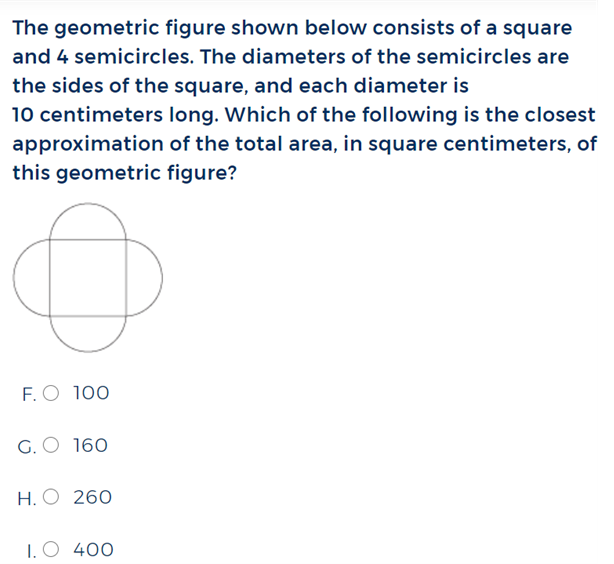Category: Geometry

Here’s how to solve it:

• Let’s begin by looking at the information we were given:

Diameter of circle: $$10$$

Radius of circle: $$5$$

Side of square (which is equivalent to the circle’s diameter): $$10$$

This key information will allow us to calculate the respective areas of the square and

• Semicircles: The area of two semicircles is equivalent to the area of one whole circle, so instead of going into the trouble of calculating four semicircle areas, we can just find the area of two whole circles.

$$2(\pi\:r^2)$$

$$2(\pi(5^2))$$

$$50\pi$$, or $$157.08$$

• Square: $$10^2=100$$

• Final Sum: $$100 + 157.08 = 257.08$$, or approximately $$260$$.

### Question 8:Category: Geometry

Here’s how to solve it:

• We can find the length of $$\bar{AG}$$ by using the distance formula to find the distance between $$A$$ and $$G$$, but first, we must find the coordinates of $$G$$.
• To do this, we can use our knowledge of the fact that $$G$$ is the center of the rectangle, making it the midpoint of $$\bar{BE}$$. Let’s apply the midpoint formula to $$\bar{BE}$$ to find $$G$$:

Midpoint $$\bar{BE}=(\frac{6+12}{2}),(\frac{4+0}{2})$$

Midpoint $$\bar{BE}= (9, 2)$$

$$G$$ is at $$(9,2)$$

• Now that we have both of our coordinates, we can apply the distance formula to $$A$$ and $$G$$:

$$\bar{AG} = \sqrt{(9 – 0)^2 + (2 – 0)^2)}$$

$$\bar{AG} = \sqrt{81 + 4}$$

$$\bar{AG} = \sqrt{85}$$.

Therefore, our answer is $$\sqrt{85}$$, or H.

### Question 9: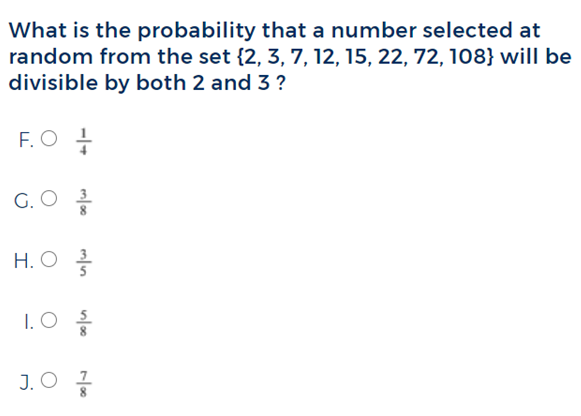Category: Statistics and Probability

Here’s how to solve it:

• This problem has a few tedious steps, but the overall concept is rather straightforward. We begin by going through every number in the set and counting how many are divisible by both 2 and 3.

Divisible by both 2 and 3: 12, 72, 108.

Not divisible by both 2 and 3: 2, 3, 7, 15, 22.

• Out of the 8 numbers presented, 3 are divisible by both 2 and 3, so that leaves us with $$\frac{3}{8}$$ as our answer!

### Question 10: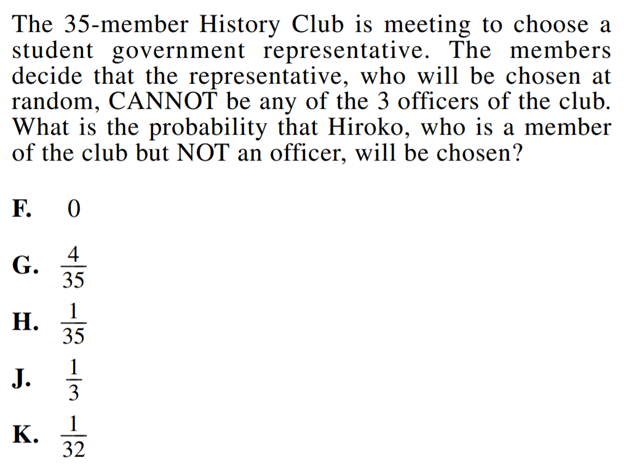Category: Statistics and Probability

Here’s how to solve it:

• Here, all we have to do is pick the correct numerator and denominator to work with. Since we are determining the probability of ONE non-officer student getting picked out of 32 (not 35, as the three officers are excluded from the raffle), Hiroko’s probability of getting picked is $$\frac{1}{32}$$.

• Keep in mind that solving most Statistics and Probability problems is just a matter of paying attention to detail and avoiding careless mistakes. Students who are moving too quickly or not paying attention might select F, for example, if they misread the question as stating that Hiroko is an officer, or H if they neglect to exclude the officers from the raffle. Read carefully!

### Question 11: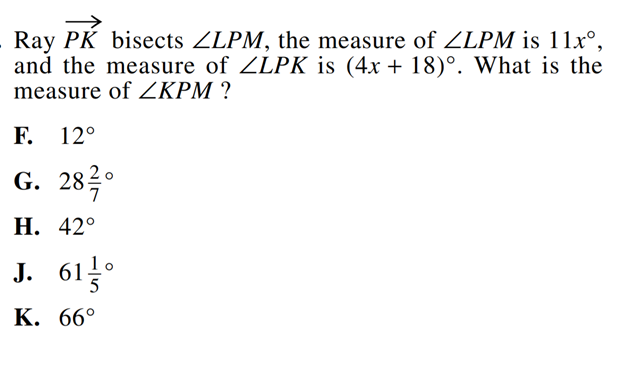Category: Integrating Essential Skills

Here’s how to solve it:

• If it helps, you can start off by drawing a picture to help yourself visualize the problem. This will help you clearly see that the measure of $$\angle{LPK}$$, when added to $$\angle{KPM}$$, is equal to the greatest angle, $$\angle{LPK}$$. Since $$\overrightarrow{PK}$$ is a bisector, we know that angles $$\angle{LPK}$$ and $$\angle{KPM}$$ are equal. This lends itself to the following equation: $$4x+18=\frac{11x}{2}$$

• We can then solve this equation to find the value of $$x$$:

$$4x+18=\frac{11x}{2}$$

$$8x + 36=11x$$

$$36=3x$$

$$12=x$$

• Since we now know that $$x=12$$, we can plug it in to the equation for $$\angle{LPK}$$, as we know it will be the same as our desired answer for $$\angle{KPM}$$:

$$\angle{KPM}=4(12)+18$$

$$\angle{KPM}=48+18$$

$$\angle{KPM}=66$$

Therefore, the correct answer is $$66^{\circ}$$, or K.

### Question 12:Category: Integrating Essential Skills

Here’s how to solve it:

• Unlike the SAT, the ACT does not provide you with a list of formulas at the beginning of the test, so it’s best to have them memorized (here’s our list of must-know ACT math formulas). Here, we will create a formula which will essentially subtract the volume of the cylinder from the volume of the cube/box. The difference will be the volume of packing material

• First, let’s find the volume of the box. Since the side lengths are $$18$$ inches, we know that the volume is $$18^3$$.

• Next, we’ll find the volume of the cylinder, $$\pi\:r^2h$$. We will plug in a radius of $$6$$ inches and height of $$12$$ inches to get $$\pi\:6^2(12)$$

• Next comes the final step of creating an equation of the two volume’s differences: $$18^3 – \pi(6)^2(12)$$

As you can see, our formula matches that of answer J.

### Question 13: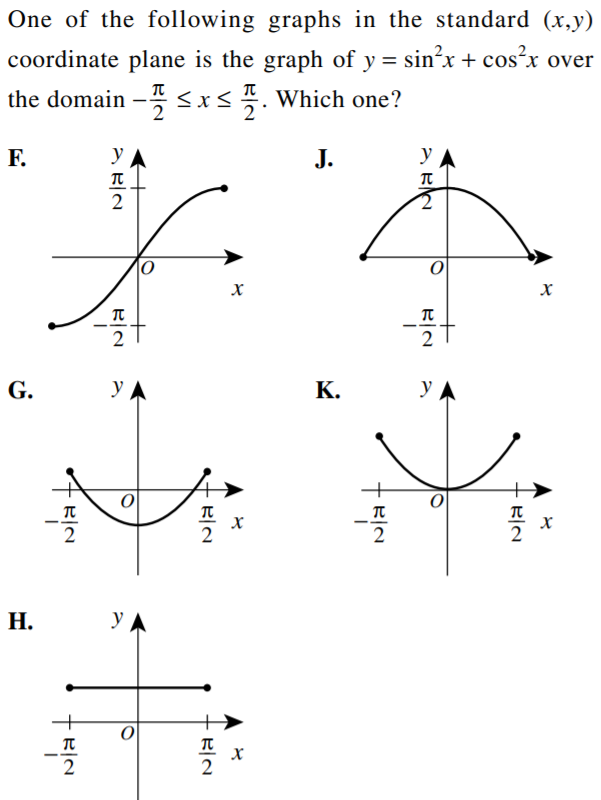Category: Modeling

Here’s how to solve it:

$$sin^2(x) + cos^2(x)$$ is actually a trig identity that always equals $$1$$. If you recognize this right off the bat, the answer is clearly H, as it’s the only constant graph. If you didn’t know this trig identity though, here’s how you’d solve this problem.

• “Domain” refers to all possible input values, i.e. all $$x$$ values. We can immediately eliminate answers F and J because they have $$-\frac{\pi}{2}$$ and $$\frac{\pi}{2}$$ as their range values, with no listed domain.

• Next, consider the fact that in radians, $$\pi = 180^{\circ}$$. That means that the domain covers the values between $$-90^{\circ}$$ and $$90^{\circ}$$. We can now plug in a few key values to figure out the value of y for the key points of $$x = -90^{\circ}$$, $$x=0$$, and $$x = -90^{\circ}$$. This can help us understand the general shape of the graph so we can select the correct answer.

• If you have the unit circle memorized, you can directly plug in the radians to get the values you need. The $$y$$-values represent sine and the $$x$$-yalues represent cosine.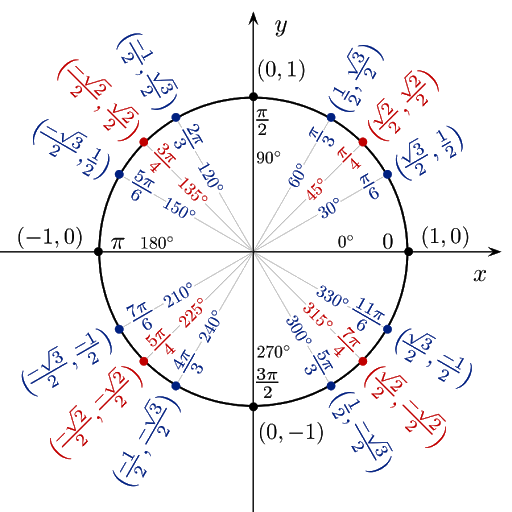• Plugging these values into $$y=sin^2(x) + cos^2(x)$$, we can understand the following information:

$$y= sin^2(-90^{\circ}) + cos^2 (-90^{\circ})$$

$$=(-1)^2+0^2$$

$$y=1$$ when $$x=\frac{-\pi}{2}$$ or $$-90^{\circ}$$

$$y= sin^2(0) + cos^2 (0)$$

$$=0^2+1^2$$

$$y = 1$$ when $$x = 0$$

$$y= sin^2(90^{\circ}) + cos^2(90^{\circ})$$

$$=1^2+0^2$$

$$y = 1$$ when $$x = \frac{\pi}{2}$$ or $$90^{\circ}$$

• Therefore, for all values of $$x$$ between $$\frac{-\pi}{2}$$ and $$\frac{-\pi}{2}$$, $$y=1$$. Since answers G and K contain different $$y$$ values for $$x$$ values of $$-90^{\circ}$$, $$0^{\circ}$$, and $$90^{\circ}$$, we know those can’t be the answer. That leaves us with our final choice, Graph H.

### Question 14:Category: Modeling

Here’s how to solve it:

• We are looking for the negative version of a graph, which is just the original graph reflected over the $$x$$ axis. Visually, we can immediately dismiss answers H, I, and J, as their slopes don’t match the original graph at all and clearly aren’t the reflected version of it.

• Choosing between answers F and G may require a closer look. Pay attention to the dashed line; it represents the graph’s limits. Since we are looking for a graph which is identical to the original aside from a reflection over the $$x$$ axis, the graph’s limit will stay in place, as it represents a consistent $$y$$ value which will stay unchanged. Therefore, our answer is F, as its limit is in quadrants 1 and 4, matching the original graph.

### Question 15:Category: Functions

Here’s how to solve it:

• As we discussed in question 13, $$\pi = 180^{\circ}$$ in radian form, so this graph will hold values within the domain of $$[-90^{\circ}, 90^{\circ}]$$. Since you will be allowed to bring your calculator to the ACT, it will be helpful to use it here, or you can again immediately plug in numbers if you have the unit circle memorized.

• As we look through the five potential answers, we know that in radian form, $$\frac{\pi}{2}= 90^{\circ}$$, and $$sin(\frac{\pi}{2})=1$$.

Let’s start off by looking at Answer option A:

$$\{\frac{-\pi}{2},\frac{\pi}{2}\}$$

$$|sin(\frac{\pi}{2})|\:\geq\:1$$ is True, because $$sin(\frac{\pi}{2})=1$$.

$$|sin(\frac{-\pi}{2})|\:\geq\:1$$ is True, because $$sin(\frac{-\pi}{2})=-1$$, and since we are looking for the equation’s absolute value here, the final answer is $$1$$.

A seems to be right, but couldn’t D also be correct? In this case, you can plug in different values in the set $$(-90^{\circ}, 90^{\circ})$$. You will never get $$sin(\theta)$$ to equal $$1$$ unless $$x=\pm\:90^{\circ}$$.

Why? Think of what sine and cosine actually represent. Let’s take a right triangle on the unit circle. The sine would be the ratio of the perpendicular (opposite) over the hypotenuse, and the cosine would be the base (adjacent) divided by the hypotenuse. So sine is represented by the $$y$$-value, and cosine by the $$x$$-value.

The perpendicular is shorter than the hypotenuse by definition, so this ratio is always less than 1 unless $$\theta= -90^{\circ}$$ or $$90^{\circ}$$ in the graph below. At $$\theta=90^{\circ}$$, $$(a, b)$$ would be at $$(0,1)$$, and we would have a straight line instead of a triangle. This is similar to $$\theta=-90^{\circ}$$, where $$(a, b)$$ would be at $$(0,-1)$$.So, we can eliminate D because $$|sin(\theta)|\:\geq\:1$$ only for $$\{\frac{-\pi}{2},\frac{\pi}{2}\}$$

## Final Tips

While studying, we recommend focusing on the topics which challenge you the most. Through building competence across all subject areas, you’ll walk into the test with the confidence needed to succeed.

If you’d like to learn more about how to do well on the ACT, you may some of our other posts:

31 ACT Math Formulas You Must Know

A Guide to the Math Section of the ACT

How to Register for the ACT + Free Resources

A Guide to the Reading Section of the ACT

Links to all the Official ACT Practice Tests + Other ResourcesMichelle Foley
Essay Breakdown Writer

Short Bio
Michelle Foley is currently taking a gap year before starting at Yale College in Fall '21, where she is considering majoring in Art, English, or Cognitive Studies while earning her Spanish certificate. In her free time, she likes to paint, run, and read!